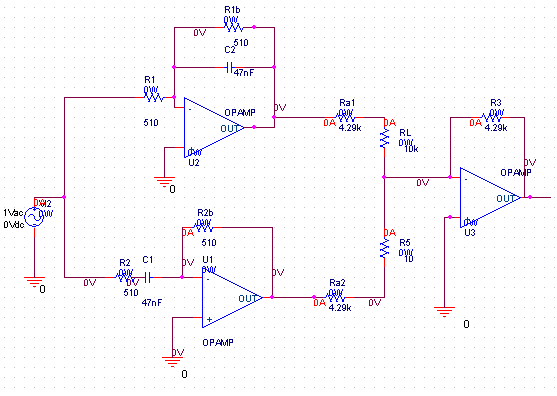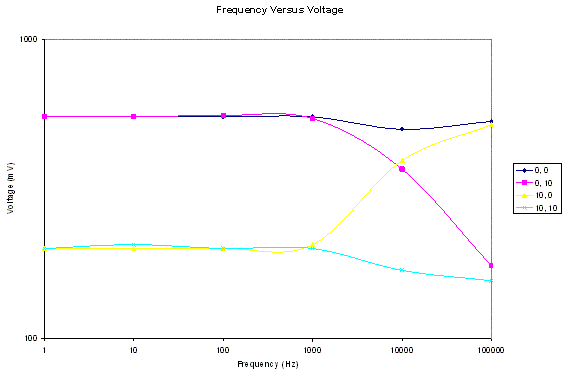Peter Lau

Bill Chun Wai Hung

Lab 7 Report ¨C Tone Controller (Amplified)

19 July 2004

A. Describe the Set-up

The setup is shown in figure 1.Figure 1

B. Desbribe Inputs

Ra = 4.29k

R3=4.29k

R1 = 510

C1 = 47n

R2 = 510

C2 = 47n

Pots are 0 to 10k ohm each.

The Op Amp used is LM 6482.

Stimuli: The Voltage is 300mV in amplitude, and the value equals to 600 mV peak-to-peak voltage.

C. Describe What you Observe

The circuit generated an experimental voltage values shown in Table 1 and Figure 3.

Table 1.

 Frequency (Hz) Voltage (mV) Setup (LP Resistance (kohm), HP Resistance (kohm)) 1 550 0,0 10 550 100 550 1000 550 10000 500 100000 531 1 550 0,10 10 550 100 556 1000 543 10000 368 100000 175 1 200 10, 0 10 200 100 200 1000 206 10000 393 100000 518 1 200 10, 10 10 206 100 200 1000 200 10000 169 100000 156Figure 3

D. What you can deduce

This circuit consists of high pass filter and low pass filter combined using a mixer circuit. This allows the frequency response to be adjusted according to the setup of the pots (0k and 0k, 0k and 10k, 10k and 0k, and 10k and 0k). The different setup give the original voltage (using 0k for the pot) or a lower voltage (30% using 10k for the pot).

What I am planning to do in order to claim my circuit works:

When the pots are adjusted, the frequency of the response change accordingly. For example, if the low pass resistance is set to zero and the high pass resistance is set to 10k, the circuit exhibits the behavior of a low pass filter. The high pass behavior can be achieved by setting low pass resistance is set to 10k and the high pass resistance is set to zero. If both high pass and low pass resistances are the same, the response should be flat. This is the result of this experiment.

The cutoff frequencies (4.66kHz)

This experiment shows the differences between ideal case and the real case.

Q6) Is the frequency response flat for both pots equal to zero?

In ideal case, the response is flat if the two filters are weighted equally. In the experimental case, at the cutoff frequency (4.66kHz) the voltage has a little dip because the low pass cutoff and the high pass cutoff does not coincide exactly. This gives the drop around the cutoff point (4.66kHz).

Q7) If it is, why? If it is not explain why and also explain what changes would you do in order to make it flat.

It is flat because at low  frequency, the output of the low pass is unattenuated. Similarly, at high  frequency, the output of the high pass is unattenuated. When these two inputs are combined, a close approximation of a flat plot can be achieved.

In the experimental case, since the cutoff frequencies (low pass and high pass cutoff frequencies) don¡¯t coincide exactly, there¡¯s a little dip. In order to fix this problem, the cutoff frequency of the high pass filter can be set a little bit lower than that of the low pass filter.